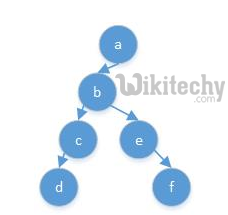# [Solved-1 Solution] How to handle recursive hierarchy in pig ?

## What is recursive function

• A recursive function (DEF) is a function which either calls itself or is in a potential cycle of function calls. As the definition specifies, there are two types of recursive functions. Consider a function which calls itself: we call this type of recursion immediate recursion.

## Problem:

• This below model have unbalanced tree data stored in tabular format like:Learn Apache pig - Apache pig tutorial - Recursive Function - Apache pig examples - Apache pig programs

• How to flatten this hierarchy where each row contains entire path from leaf node to root node in a row as:

How to solve above problem using hive, pig or mapreduce?

## Solution 1:

Here is the sample code to handle recursive hierarchy in pig

## Why join

• A Join simply brings together two data sets. These joins can happen in different ways in Pig - inner, outer, right, left, and outer joins. These however are simple joins and there are specialized joins supported byPig.

Using join is one of the way to handle this function